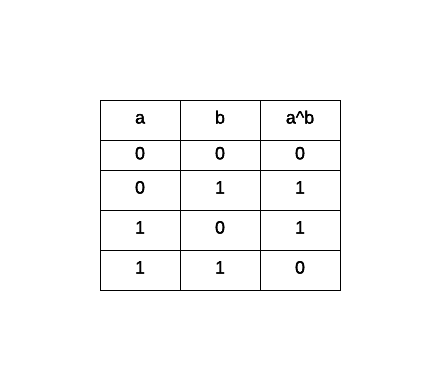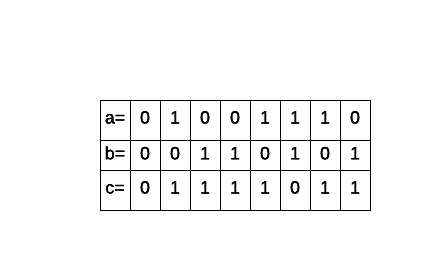# AP Computer Science A : Run Time Exceptions

## BITWISE XOR OPERATION

Given the following binary values

a = 0100 1110

b = 0011 0101

perform an XOR operation (c = a^b). What is the result?

c = 0111 1111

c = 0111 1011

c = 0000 0100

c = 0111 0011

c = 0111 1011

Explanation:

Performing a bitwise excludive OR constitutes in taking both binary values and evaluating as follows: either one or the other (1) never both (0). This is the truth table for a bitwise XOR operation:Taking both a and b and performing the operation bit by bit we get the following result:### Example Question #11 : Algorithm Analysis

True or False.

There is a runtime exception in this code snippet.

int wait_time = 0;

int wait_time = 5;

for (int i = 0; i < wait_time; i++) {

System.out.println(wait_time);

}

True

False